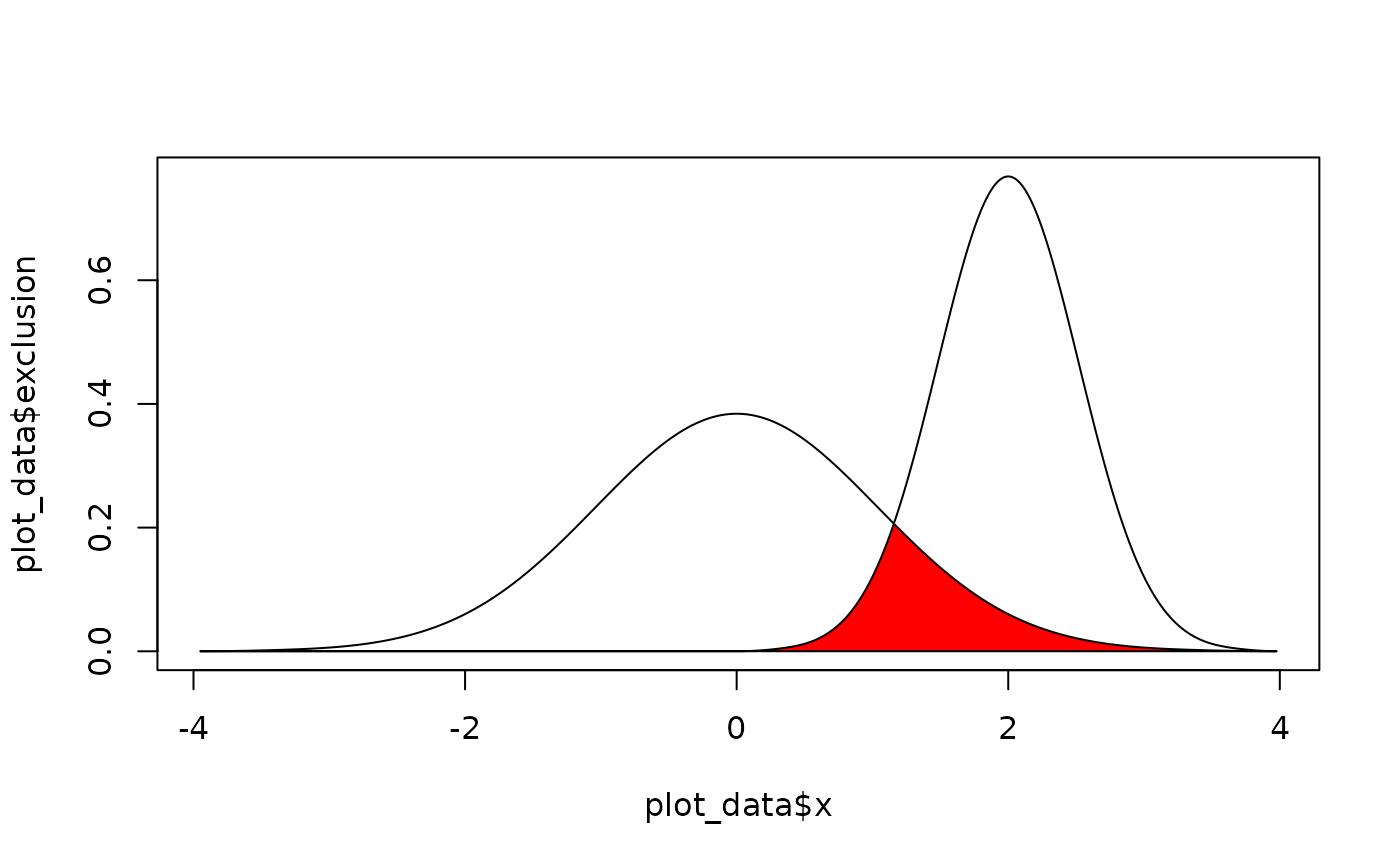A method to calculate the overlap coefficient between two empirical distributions (that can be used as a measure of similarity between two samples).

## Usage

overlap(
x,
y,
method_density = "kernel",
method_auc = "trapezoid",
precision = 2^10,
extend = TRUE,
extend_scale = 0.1,
...
)

## Arguments

x

Vector of x values.

y

Vector of x values.

method_density

Density estimation method. See estimate_density().

method_auc

Area Under the Curve (AUC) estimation method. See area_under_curve().

precision

Number of points of density data. See the n parameter in density.

extend

Extend the range of the x axis by a factor of extend_scale.

extend_scale

Ratio of range by which to extend the x axis. A value of 0.1 means that the x axis will be extended by 1/10 of the range of the data.

...

Currently not used.

## Examples

library(bayestestR)

x <- distribution_normal(1000, 2, 0.5)
y <- distribution_normal(1000, 0, 1)

overlap(x, y)
#> # Overlap
#>
#> 18.6%
plot(overlap(x, y))